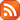Subscribe by RSS

Mon., Apr. 3, 2023 3:30 p.m.

Location: CK 185 and Live Stream

Speaker: Arfa Khalid

Title: Analyzing the effectiveness of Covid-19 vaccines among different age groups using Multinomial logistic regression Model (291 kB)Abstract:

Multinomial Logistic Regression (MLR) is a statistical modeling technique used to analyze relationships between multiple categorical variables. In this seminar, the theory and application of Multinomial Logistic Regression (MLR) in analyzing the effectiveness of Covid-19 vaccines among different age groups will be explored. Firstly, we will begin by introducing the Multinomial Random Variable and its properties, followed by the definition and components of the Multinomial Logistic Regression Model, along with the Logistic Regression Model with Covariates. Then we will talk about the Model design in depth, including multivariate distribution, its mathematical proof, and interaction effect. Specifically, we will focus on how these design components are used to analyze vaccination effectiveness across various age groups.

Live Stream:

https://uregina-ca.zoom.us/j/94125367372